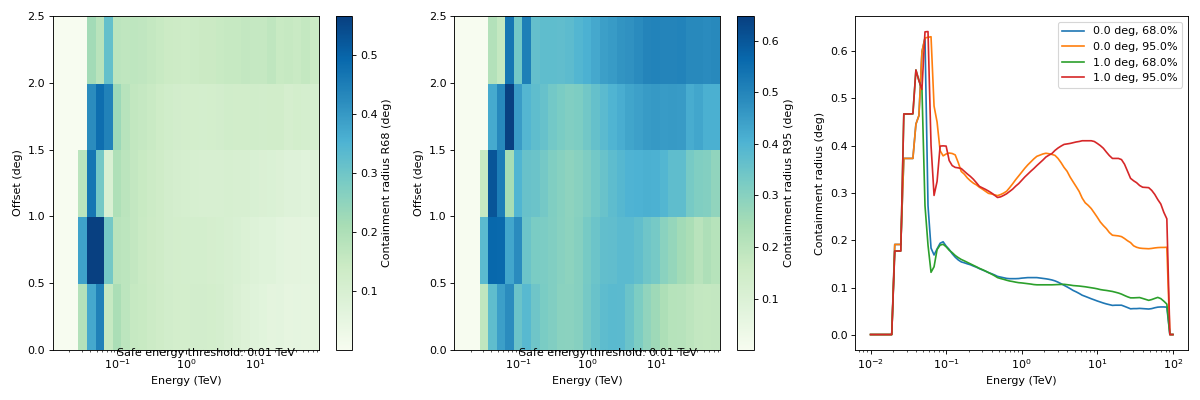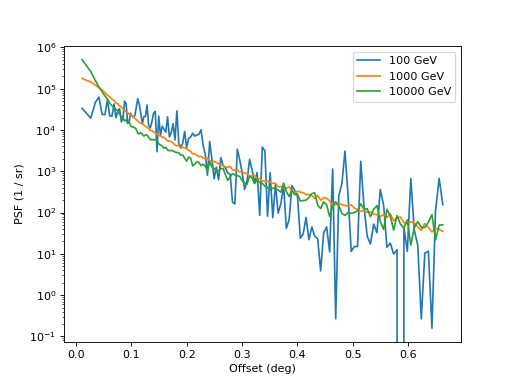## as a function of of true energy and offset angle (PSF_TABLE)¶

The PSF3D class represents the radially symmetric probability density of the angular separation between true and reconstructed directions $$\delta p = p_{\rm true} - p$$ (or rad), as a function of true energy and offset angle from the field of view center ($$PSF(E_{\rm true}, \delta p|p_{\rm true})$$ in IRF Theory).

Its format specifications are available in PSF_TABLE.

This is the format in which IACT DL3 PSFs are usually provided, as an example:

"""Plot a PSF from the HESS DL3 data release 1."""
import matplotlib.pyplot as plt
from gammapy.irf import PSF3D

filename = "$GAMMAPY_DATA/hess-dl3-dr1/data/hess_dl3_dr1_obs_id_020136.fits.gz" psf = PSF3D.read(filename, hdu="PSF") psf.peek() plt.show()  (png, hires.png, pdf)## as a function of true energy (PSF_GTPSF)¶ EnergyDependentTablePSF instead represents the probability density of the angular separation between true direction and reconstructed directions $$\delta p = p_{\rm true} - p$$ (or rad) as a function of true energy only ($$PSF(\delta p| E_{\rm true})$$ following the notation in IRF Theory). Its format specifications are available in PSF_GTPSF. Such a PSF can be obtained by evaluating the PSF3D at a given offset (using Angle) """Plot the PSF at a given offset from the camera center""" from astropy.coordinates import Angle import matplotlib.pyplot as plt from gammapy.irf import PSF3D filename = "$GAMMAPY_DATA/hess-dl3-dr1/data/hess_dl3_dr1_obs_id_020136.fits.gz"
# offset at which we want to examine the PSF
offset = Angle("0.5 deg")
psf_table = psf.to_energy_dependent_table_psf(offset)Note: the offset angle on the x axis is not an offset angle from the field of view center but the above mentioned angular separation between true and reconstructed direction $$\delta p$$ (in the IRF axis naming convention - see irf - Instrument response functions - the term rad is used for this axis).